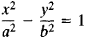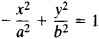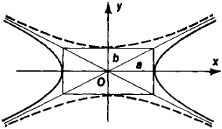Conjugate Hyperbolas

conjugate hyperbolas

[′kän·jə·gət hī′pər·bə·ləz]
(mathematics)
Two hyperbolas having the same asymptotes with semiaxes interchanged.

Conjugate Hyperbolas

two hyperbolas defined in the same rectangular coordinate system by the equationsandwhere a and b have the same values in both equations. Conjugate hyperbolas have the same asymptotes. The transverse and conju-Figure 1

gate axes of one conjugate hyperbola are, respectively, the conjugate and transverse axes of the other (see Figure I).

Site: Follow: Share:
Open / Close